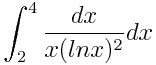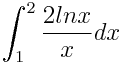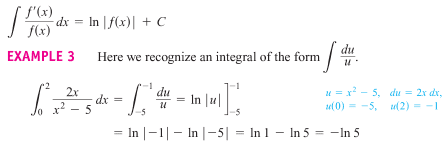# Transcendental Functions Containing Natural Logs

WhiskeyHammer

## Homework Statement

Evaluate the integrals

a) y=b) y=## Homework Equations## The Attempt at a Solution

I've tried using the Reciprocal Rule and substitution of each ln function with u to get a workable result, but haven't come anywhere near a recognizable solution. The answers stated in the book are as follows:
a) 1/ln4
b) (ln2)^2

[STRIKE]This tells me that, somehow a must end up looking like this:
ln 1 - ln 4
and b must look like:
ln2 * ln2[/STRIKE]

These would seem counter intuitive to my understanding of how these kinds of problems are solved and I am at a loss of how to fix it.

Last edited:

Homework Helper
Gold Member
You want the anti-derivative (definite integral), not the derivative, and there is no ##y## in either problem. In both problems try the substitution ##u=\ln x##.

Mentor
The answers stated in the book are as follows:
a) 1/ln4

This tells me that, somehow a must end up looking like this:
ln 1 - ln 4
1/ln(4) ≠ ln(1) - ln(4), so it would be good for you to review the properties of logs.

The expression on the right can be simplified like so:

ln(1) - ln(4) = 0 - ln(4) = ln(1/4)

Note the ln(1/4) is a negative number, while 1/ln(4) is positive, so they couldn't possibly be equal.

WhiskeyHammer
You want the anti-derivative (definite integral), not the derivative, and there is no ##y## in either problem. In both problems try the substitution ##u=\ln x##.

I see the intent in setting up the integral as ##u*du## and anti-deriving to ##2u## but I don't see how ##du## could possibly equal ##1/x*dx## the derivative of ##\ln x## is ##(1/x)(d/dx*x)## which leaves us with ##u*du*dx## right?

I also updated the errors in my original post. Thanks for catching them, I am definitely on the tail end of burned out.

Mentor
I see the intent in setting up the integral as ##u*du## and anti-deriving to ##2u## but I don't see how ##du## could possibly equal ##1/x*dx## the derivative of ##\ln x## is ##(1/x)(d/dx*x)## which leaves us with ##u*du*dx## right?
No. If u = lnx, then du = d(lnx) = d/dx(lnx)*dx = 1/x * dx
I also updated the errors in my original post. Thanks for catching them, I am definitely on the tail end of burned out.

Homework Helper
I see the intent in setting up the integral as ##u*du## and anti-deriving to ##2u## but
Ouch! The anti-derivative of u is $(1/2)u^2+ C$

I don't see how ##du## could possibly equal ##1/x*dx## the derivative of ##\ln x## is ##(1/x)(d/dx*x)## which leaves us with ##u*du*dx## right?

I also updated the errors in my original post. Thanks for catching them, I am definitely on the tail end of burned out.

$$\int \frac{dx}{x ln(x)}$$
If you let u= ln(x) then $du= \frac{1}{x}dx= \frac{dx}{x}$ so the integral becomes
$$\int\frac{dx}{x ln(x)}= \int \frac{1}{ln(x)}\frac{dx}{x}= \int \frac{1}{u}du$$
Thanks guys, despite the errors on my part, you suggestions and clarifications were awesome and I got it worked out